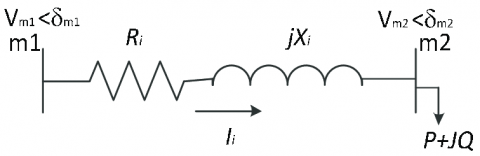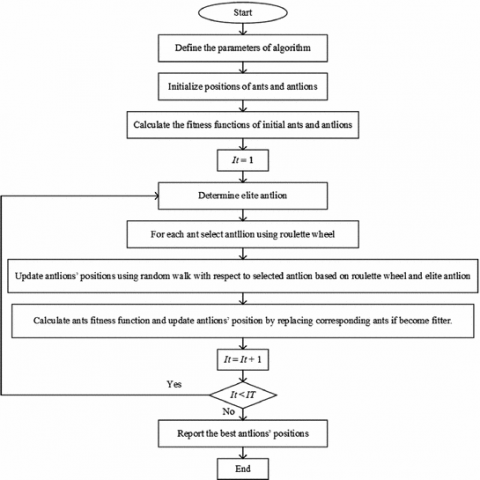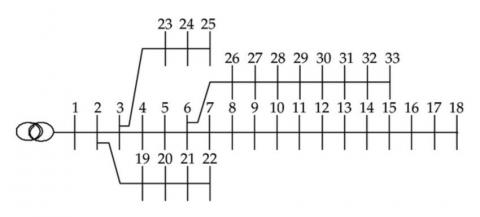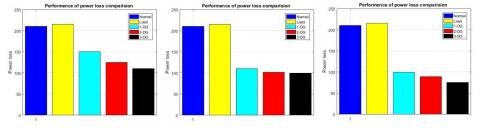# Multi distributed generation placement using ant-lion optimization

Multi distributed generation placement using ant-lion optimization

Rajesh Kumar SamalaMercy Rosalina Kotaputi

Vignan's Foundation for Science, Technology and Research, Dept. of Electrical and Electronics Engineering, Vadlamudi, India

Corresponding Author Email:
rajeshkumar_samala@yahoo.co.in
Page:
253-267
|
DOI:
https://doi.org/10.3166/EJEE.19.253-267
|
Accepted:
|
Published:
31 December 2017
| Citation

OPEN ACCESS

Abstract:

This paper deals an advanced technique for Distributed Generation (DG) location at multiple places with optimal size in radial distribution system to enhance the voltage at every bus and active power minimization. Initially the BackWard and ForWard (BW/FW) sweep analysis is using to fetch the values of individual bus voltages and respective angles, reactive and active power loss at every bus. The DG location in the system is determine by finding Voltage Stability Index (VSI) value and the capacity of DG is decide by an objective function using different algorithms. The research on IEEE-33 bus on MATLAB platform is presented. A comparison analysis is also presented to prove that the voltage at every bus enhancement and active power loss reduction with the proposed algorithm.

Keywords:

distributed generation, backward and forward sweep method, ant-loin optimization algorithm. optimal capacity, optimal place, active power loss.

1. Introduction

Distributed Generations (DG) will offer many solutions for different consumers those are much cost-effective, efficiency is high, operating at very low cost of fuel, much eco-friendly, very high power quality, reliability than the traditional generations. DGs can available in the range of 5 kW to 5 MW which include: Reciprocating Engines (5-500kW), Micro-turbines (30-200kW), Industrial Combustion turbines (1MW-5MW), Fuel Cells (5-1000+kW), Photovoltaic (less than 5kW), Wind turbine (5-1000kW). Among all benefits DGs will be used by customers for expand the available system and to avoid the transmission and distribution losses.

Many methodologies available for getting optimal capacity and placing of DGs in radial systems (Kansal et al., 2014). The issues must be consider for integrating DGs in to the existing system are: number of DGs and their location and size, the method is used to determine the optimal location, total loss reduction after DG installation in the system. Various techniques proposed based on analytical approaches, optimization methods or heuristic methods. Many of them are to determine the best place and capacity of one DG in order to enhance the voltage at every bus and minimize active power losses (Abu-Mouti and El-Hawary, 2011; Ochoa et al., 2006; Imrana et al., 2014). Some of them discuss the multi DGs placement with various optimization techniques.

Mardaneh and Gharehpetian (2004) have presented a Genetic Algorithm (GA) based approach with Optimal Power Flow (OPF) measurements to obtain optimal place and capacity of DG to integrate in to the system.

Wang et al. (2017) have presented the operation features for long-term for DG; a mixed integer non-linear optimization issue was expressed to perfectly regulate for sizing and siting of Soft Open Points (SOPs) based on distinctive procedure situations produced by Wasserstein space. Later that was malformed and resolved as a mixed integer second-order programming scheme. The SOPs comes under power electronic components fitted to substitute generally open nodes in electrical distribution schemes. SOPs could give reactive/real power flow regulation and control of voltage when normal situations and also isolation of fast fault and restoration of supply under abnormal circumstances. The implementation of SOPs could progress the distribution schemes controllability, therefore more augments the economy, reliability and flexibility of the grid.

Mokgonyana et al. have proposed a development scheme for electricity distribution corporations (DISCOs) for improving revenue. The scheme regulates optimum size and place for non-conventional sources of energy, they were considered as Non-dependent Electricity Generation (NEG). NEG denotes to investors of third party owned generators and connected to quota obligation machinery. Self-generation includes low capacity generators are reinforced by tariffs of feed-in which delivery energy for local consumption, transferring excess generation to the system. The attained best planning scheme was able to assess system size for revenue maximization if the DISCO was gratified to give system access to self-generation and NEG. Different quantities of the objective function, owed to the explanation of self-generation, are profit erosion, retrieval and also the excess energy cost.

Dinakara Prasad Reddy et al. (2016) have proposed a flower pollination optimization algorithm for finding the best capacity of DG. DG sources were small, self-contained electric generating plants that could provide power to homes, businesses or industrial facilities in distribution feeders. The optimally locating DG could minimize loss of power and voltage profile improvement. However, the DGs values were mostly dependent on their types, capacities and places as they were installed in distribution feeders. The main contribution was to determine the best places for DG units and capacity. Index vector method was utilized for best DG places and utilises 3-different types of DG units for compensation.

Saha and Mukherjee (2016) have projected Chaotic Symbiotic Organisms Search (CSOS) approach to detect the best size and location of DGs in an RDS with constant load models. DGs show an imperative character to diminish the active power loss and to progress the power system voltage stability. This DG sitting and capacity issue is related to active power loss minimization and voltage profile enhancement. Though, best place and capacity of DGs in the radial network is an important problem, improper siting and capacity of DGs should upsurge the overall system loss.

Abbasi and Hosseini (2016) have projected a Distribution System Reconfiguration (DSR), in view of system formation result which runs in offline method at continuous loads and optimal DG distribution and capacity issues are considered concurrently to detect a better situation for radial system on the basis of working thresholds and reliability enhancements. The technique of calculating energy not supplied in DSR issue in the DGs availability with storage schemes was elucidated and protective equipment effect was measured as well. Power systems optimal performance significantly depends on system configuration, capacity and place DGs storage systems and units.

Babacan et al. (2017) have presented Energy Storage Schemes of Battery (ESSB) in radial networks for support of mitigation of voltage by integrating solar PV. A bi-level optimization method based on GA was established that minimizes the variations of voltage due to connection of PV through arranging ESSB among a radial network acceptable nodes when accounting for their investment and connection cost utilizing a qualitative cost scheme. Optimization issue considers ESSB size and locating nodes in radial network as decision variables. Every ESSB process was found by utilizing Linear Programming (LP) routine which reduces the day-to-day equivalent highest demand.

Hadidian-Moghaddam et al. (2017) have projected an optimization method is to explain the best location and size of DG in a Radial Distribution System (RDS). The issue of optimization was answered by using an ALO by considering various objectives. These objectives are purchased energy cost reduction from upstream system, Improvement of reliability; DGs cost reduction, RS loss reduction and bus voltage deviation reduction. This issue was solved as a Multi-Objective Optimization (MOO).

Das et al. were presented reconfiguration of system radial system reconfiguration with DGs presence by having different bus kinds those are P bus as well as PQV bus. ‘P’ bus was characterized by only active power specification. However the PQV voltage at bus was controlled remotely by the P bus. A technique was projected for selection of bus P for magnitude of voltage control located PQV bus remotely. An analysis of sensitivity method was utilized to select the buses for DGs location for working at power factor of unity. DGs location was estimated in sequential placement and non-sequential placement. GA methodology was utilized for the DGs optimization followed by system reconfiguration.

Lot of research has done by considering power factor as unity to locate DG. This work enables to decide the best place and capacity of DGs at multiple locations by injecting active power in order to obtain the objective function. Finally, the enhancement of voltages at every bus and minimization of system active power losses using an advanced technique is inspected and all the outcomes are evaluate with conventional techniques.

2. Problem formulation

This paper the mainly concentrate on improvement of bus voltages and minimization of active power loss in distribution network utilizing one DG, multi DG system. By using BW/FW sweep analysis initially, calculate system reactive and active power loss and voltages at every bus. Then the reduction of power loss indices also with per unit bus voltages, which are considered as, inputs for an advanced algorithm, which determine the best DG place and size.

2.1. Objective functions

## 1.pngFigure 1. Two bus single line diagram

The above Figure 1 represents a two-bus radial network. This paper deals the issue of active power loss minimization and improvement of voltage at all the buses in the test system after and before integrating DGs in best sites with proper capacity. The main objective function is to enhance the voltage profile and the active power loss reducing as follows:

F=\left\{ \begin{align} & \min .active\text{ }power\text{ }loss\text{ }({{f}_{1}}) \\ & \min .Voltage\text{ }deviation\text{ }at\text{ }every\text{ }bus\text{ }({{f}_{2}}) \\ & \max .Voltage\text{ }Stability\text{ }Index\text{ }(VSI)\text{ }({{f}_{3}}) \\ \end{align} \right.    (1)

The mathematical formula for calculating power loss in the network is (Elgerd and Happ, 1971),

$\min active\text{ }power\text{ }loss\text{ }\left( {{f}_{1}} \right)=\min \left( {{P}_{L}} \right)$      (2)

Where, ${{P}_{L}}=\sum\limits_{i=1}^{N}{\sum\limits_{j=1}^{n}{\left[ {{\alpha }_{ij}}\left( {{P}_{i}}{{P}_{j}}+{{Q}_{i}}{{Q}_{j}} \right)+{{\beta }_{ij}}\left( {{Q}_{i}}{{P}_{j}}-{{P}_{i}}{{Q}_{j}} \right) \right]}}$, ${{\alpha }_{ij}}=\frac{{{r}_{ij}}}{{{V}_{i}}{{V}_{j}}}\cos \left( {{\delta }_{i}}-{{\delta }_{j}} \right)$, ${{\beta }_{ij}}=\frac{{{r}_{ij}}}{{{V}_{i}}{{V}_{j}}}\sin \left( {{\delta }_{i}}-{{\delta }_{j}} \right)$ and ${{Z}_{ij}}={{r}_{ij}}+j{{x}_{ij}}$ is the ijth element of [Zbus] matrix, N–Total bus numbers.

Another objective function is to improve the system bus voltages, mathematically represented as:

$\min Voltage\text{ }deviation\left( {{f}_{2}} \right)=\sum\limits_{ni=1}^{{{N}_{n}}}{{{\left( {{V}_{ni}}-{{V}_{rated}} \right)}^{2}}}$   (3)

Where, Nn is the number of system buses, Vni specifies the ith bus voltage and Vrated specifies the rated bus voltage of the network that is equal to1.0 p.u.

The final objective function in this paper is Voltage Stability Index (VSI) is represented as follows:

$\max (VSI(ni))={{\left| {{V}_{mi}} \right|}^{4}}-4\left[ {{P}_{ni}}(ni){{R}_{ni}}+{{Q}_{ni}}(ni){{X}_{ni}} \right]{{\left| {{V}_{mi}} \right|}^{2}}- 4{{\left[ {{P}_{ni}}(ni){{R}_{ni}}+{{Q}_{ni}}(ni){{X}_{ni}} \right]}^{2}}$    (4)

Where, Pni(ni) is Total active power fed through bus ni, Qni(ni) is Total reactive power through bus ni, Rni is i-th branch resistance, Xni is i-th branch reactance and SI(ni) is VSI (Voltage stability Index) of ni node.

${{P}_{i}}={{P}_{Gi}}-{{P}_{Di}}$    (5)

${{Q}_{i}}={{Q}_{Gi}}-{{Q}_{Di}}$     (6)

Where, PGiand QGispecifies real and reactive generated power at bus i respectively; PDiand QDispecifies real and reactive demand of load at bus i respectively.

2.2. Constraints

2.2.1. Voltage

The deviation of voltage is specified in the limits of 0.9 p.u. and 1.05 p.u.

${{V}_{i,\min }}\le {{V}_{i}}\le {{V}_{i,\max }}$        (7)

Where, Vmin is minimum voltage at bus i, Vi is the voltage at bus i and Vmax is the maximum voltage at bus i.

2.2.2. Power

$P{{L}_{afterDG}}\le P{{L}_{beforeDG}}$    (8)

Where, PLafterDG is the Power Loss after DG installation and PLbeforeDG is the Power Loss before DG installation.

2.2.3. Distributed generation

$\sum\limits_{i=1}^{Nbus}{0\le {{P}_{DG}}\le {{P}_{Di}}}$    (9)

$\sum\limits_{i=1}^{Nbus}{0\le {{Q}_{DG}}\le {{Q}_{Di}}}$     (10)

Where, PDGand QDGare the active and reactive injected by DG at the same bus i, PDiand QDiare the active and reactive load demand at the same bus i.

3. Analysis of power flow

Initially for power flow analysis the Backward/Forward sweep analysis is utilized. In this power flow analysis, firstly the currents in every branch is calculated starting from end node to the reference node using backward sweep process. Secondly, forward analysis takes place initiates from the reference node to the terminal node. In forward sweep analysis, all the bus voltages are calculated by using currents obtained in backward sweep process and verify for tolerance. The base voltage of the system is 12.66 KV and the base MVA is 100.Tolerance value is 0.0001p.u.

## 2.jpgFigure 2. Backward and forward sweep analysis flowchart

4. The ALO algorithm for optimal locating and capacity the DG

In 2015 Mirjalili was presented an Ant-Lion Optimizer (ALO) which was inspired by nature (Raju et al., 2016). ALO emulates hunting behaviour of ant-lion’s from nature. The important two phases in ALO life cycle are Larvae phase and adult phases. Larvae phase of Ant lions for hunt and reproduction in adult phase. Larvae phase is the motivation for ALO algorithm. ALO algorithm has mainly five operations those are ants’ movement randomly, trap building, ants’ trapping in traps, catching of food/prays and re-building of traps. Ants’ positions randomly are stored in the matrix Mant is given as equation (11):

${{M}_{ant}}=\left( \begin{matrix} An{{t}_{1,1}} & An{{t}_{1,2}} & \ldots & An{{t}_{1,n}} \\ An{{t}_{2,1}} & An{{t}_{2,2}} & \ldots & An{{t}_{2,d}} \\ \vdots & \vdots & \vdots & \vdots \\ An{{t}_{n,1}} & An{{t}_{n,2}} & \ldots & An{{t}_{n,d}} \\\end{matrix} \right)$       (11)

Here Am,n describes the mth value of variable of nth ant, n denotes the ants number (population size). Ant’s fitness should be kept within the MOA matrix in terms of the objective function f is given as equation (12):

${{M}_{OA}}=\left( \begin{matrix} f\left[ An{{t}_{1,1}},An{{t}_{1,2}},\ldots ,An{{t}_{1,n}} \right] \\ f\left[ An{{t}_{2,1}},An{{t}_{2,2}},\ldots ,An{{t}_{2,d}} \right] \\ \vdots \\ f\left[ An{{t}_{n,1}},An{{t}_{n,2}},\ldots ,An{{t}_{n,d}} \right] \\\end{matrix} \right)$   (12)

The location and fitness of ant-lion are shown by the matrices Mantlion and MOAL, which is given as equation (13):

${{\text{M}}_{\text{antlion}}}\text{=}{{\text{M}}_{\text{OAL}}}\text{=}\left( \begin{matrix} \text{f}\left( \left[ \text{A}{{\text{L}}_{\text{1,1}}}\text{,A}{{\text{L}}_{\text{1,2}}}\text{,,A}{{\text{L}}_{\text{1,n}}} \right] \right) \\ \text{f}\left( \left[ \text{A}{{\text{L}}_{\text{2,1}}}\text{,A}{{\text{L}}_{\text{2,2}}}\text{,,A}{{\text{L}}_{\text{2,n}}} \right] \right) \\ \text{M} \\ \text{f}\left( \left[ \text{A}{{\text{L}}_{\text{n,1}}}\text{,A}{{\text{L}}_{\text{n,2}}}\text{,,A}{{\text{L}}_{\text{n,n}}} \right] \right) \\\end{matrix} \right)$   (13)

4.1. Ants movement randomly

ALO approach mimics the interaction among ants and ant-lions in the trap. To describe this interaction, ants should move along the search area and ant-lions were permitted to hunt ants and become best fitter utilizing traps. All ants move randomly for food search. Ants’ random movement is shown by equation (14):

$X(t)=\left[ 0,cummsum(2r({{t}_{1}})-1),cummsum(2r({{t}_{2}})-1),...,cummsum(2r({{t}_{n}})-1) \right]$  (14)

Here cummsum is cummulative sum, n signifies as number of ants, t described as iteration and r(t) denotes the random number generation, rand signifies a random number created with constant distribution in [0,1], which given as equation (15):

$r(t)=\left\{ \begin{matrix} 01\,\,\,when\text{ }rand>0.50 \\ 00\,\,\,when\,rand<0.50 \\\end{matrix} \right.$       (15)

Normalized form is utilized to confine the arbitrary movement within the search area. Location of ants will be changed by equation (16):

$X_{m}^{t}=\frac{(X_{m}^{t}-{{a}_{m}})({{b}_{m}}-c_{m}^{t})}{dd_{m}^{t}-{{a}_{m}}}+{{c}_{t}}$      (16)

Here am and bm are ants random walk minimum and maximum, ctm and ddtm are mth variable at tth iteration minimum and maximum.

4.2. Trapping of ants

Ant’s random walk is affected by traps constructed by ant-lion’s traps. Ants trapping expression into the ant-lion’s pits is mathematically represented by equations (17) and (18):

$c_{m}^{t}=Ant-lion_{n}^{t}+{{c}^{t}}$         (17)

$dd_{m}^{t}=Ant-lion_{n}^{t}+d{{d}^{t}}$       (18)

4.3. Trap construction

By using Roulette wheel technique the fittest ant-lion is selected during iterations used in ALO. This mechanism gives high opportunities for ants catching.

4.4. Ants sliding into pits

Relative to fitness of ant lions they can prepare traps and ants were assumed to randomly move. Ant lions shoot sands away from the pit centre when an ant-lion recognises that there is an ant inside trap. This behaviour slips down the trapped ant when is trying to escape. For describe this behaviour, the area of random walk of ant's hyper sphere is adaptively condensed. Ants slipping into pits are represented by equations (19) and (20):

${{c}^{t}}=\frac{{{c}^{t}}}{I}$      (19)

$d{{d}^{t}}=\frac{d{{d}^{t}}}{I}$     (20)

Here I=10.0w t/s, t specifies present iteration, S specifies the maximum iterations and w specifies a constant. The w measurement is described by equation (21):

$w=\left\{ \begin{matrix} 02\,\,\,\,when\text{ }\,t>0.10S \\ 03\,\,\,\,when\text{ }\,t>0.50S \\ 04\,\,\,\,when\text{ }\,t>0.750S \\ 05\,\,\,\,when\text{ }\,t>0.90S \\ 06\,\,\,\,when\text{ }\,t>0.950S \\\end{matrix} \right.$    (21)

4.5. Pray/food catching and pit reconstruction

Than the chosen ant lion, if the ant having a perfect objective functions later it modifies their place to the new location of the hunted ant to advance its catching chance of new ant. Later, in order to catch new prey ant-lion should update the location (Rajan et al., 2017). This is given by equation (22):

$Ant\,lion_{n}^{t}=Ant_{m}^{t}\,\,if\,f(Ant_{m}^{t})>f(Ant\,lion_{j}^{t})$    (22)

4.6. Elitism

Elitism was utilized to have the finest results in each phase. Ant lion can be considered as elite when it is globally best. For this by Roulette wheel all ants were assumed to coordinate with an ant-lion and the elite is described by equation (23):

$An{{t}^{t}}=\frac{R_{A}^{t}+R_{B}^{t}}{2}$       (23)

Here RA and RB is the ant walk randomly about an ant-lion particularly and elite at iteration tth simultaneously. This represents that ant walk randomly is highly constrained around the elite ant lions and as a consequently, algorithm experiences poor exploration. The concept of meta-heuristic algorithms is extremely requires appropriate balance among exploration and exploitation (Moradi and Abedini, 2012).

## 3.pngFigure 3. ALO algorithm analysis flowchart

5. Test system

In this paper, an IEEE-33 radial distribution system has considered for examine the improvement of voltage at each bus and minimization of active power loss. This network has 33 nodes and 32 branches. The total real power demand is 3800 kW and the reactive power demand is 2690 kVAr.

## 4.jpgFigure 4. IEEE-33 radial distribution system

6. Results and discussion

Table 1. Total summary of results obtained by multi DG placement using various algorithms

 No. of DGs Method Bus No Power Loss (kW) DG size (kW) Min Voltage Without DG With DG % loss reduction after DG Without DG With DG % Voltage Improvement Without Load With load Without Load With load 1 ALO 32 210.01 215.11 99.25 52.74 53.86 2500 0.895 0.9754 8.91 BAT 32 110.29 47.48 48.72 2500 0.9525 6.35 GSA 32 150.54 28.31 30.31 2500 0.9425 5.24 2 ALO 32 89.25 57.50 58.51 1500 0.9826 9.71 5 1000 BAT 32 102.01 51.42 53.20 1500 0.9621 7.43 5 1000 GSA 32 125.12 40.42 41.83 1500 0.9522 6.32 5 1000 3 ALO 32 75.26 64.16 65.01 1500 0.9891 10.44 5 750 18 250 BAT 32 99.65 54.92 55.32 1500 0.9695 8.25 5 750 18 250 GSA 32 110.58 47.34 48.60 1500 0.9601 7.20 5 750 18 250

The percentage of power loss reduction of connected multi DGs are given in the Table 1. With 1-DG, the percentage of minimization in active power loss by using ALO is 53.86%, with 2-DGs, it is 57.50%, and with 3-DGs, it is 64.16%. It clearly understand from the outcomes that with increase in DGs number, the percentage minimization in real power loss is much effective.

Table 2. Comparison analysis of VSI

 Methods VSI (p.u) ALO 0.9847 BAT 0.9578 GSA method (Jamian et al., 2014) 0.9364 IWD (Prabha et al., 2015) 0.9155 BFOA (Mohamed and Kowsalya, 2014) 0.9047 Multi objective particle swarm optimization (MOPSO) 0.87 Particle Swarm Optimization (PSO) (Moradi and Abedini, 2012) 0.81 Genetic Algorithm (GA) 0.72

## 5.jpgFigure 5. Voltage comparisons using GSA, BAT and ALO methods individually

## 6.jpgFigure 6. Voltage comparisons using GSA, BAT and ALO methods

## 7.jpgFigure 7. Power loss comparisons using GSA, BAT and ALO methods individually

## 8.jpgFigure 8. Power loss comparisons using GSA, BAT and ALO methods

7. Conclusion

It is very clear from the results that there is an observable reduction in active power losses with 1-DG, 2-DG and 3-DG placement. The reduction in active power losses has been found by using three different algorithms. Present an impact of introducing multi DGs in to the system the voltage at all bus has been improved.

References

Abbasi F., Hosseini S. M. (2016). Optimal DG allocation and sizing in presence of storage systems considering network configuration effects in distribution systems. IET Generation, Transmission and Distribution, Vol. 10, No. 3, pp. 617-624. https://doi.org/10.1049/iet-gtd.2015.0367

Abu-Mouti F. S., El-Hawary M. E. (2011) Heuristic curve-fitted technique for distributed generation optimization in radial distribution feeder systems. IET Generation, Transmission & Distribution, Vol. 5, No. 2, pp. 172-180. https://doi.org/10.1049/iet-gtd.2009.0739

Babacan O., Torre W., Kleissl J. (2017). Siting and sizing of distributed energy storage to mitigate voltage impact by solar PV in distribution systems. An International Journal of Solar Energy, Vol. 146, pp. 199-208. https://doi.org/10.1016/j.solener.2017.02.047

Dinakara Prasad Reddy P., Veera Reddy V. C., Gowri Manohar T. (2016). Application of flower pollination algorithm for optimal placement and sizing of distributed generation in distribution systems. An International Journal of Electrical Systems and Information Technology, Vol. 3, No. 1, pp. 14-22. http://dx.doi.org/10.1016/j.jesit.2015.10.002

Elgerd I. O., Happ H. H. (1971). Electric energy system theory: An introduction. IEEE Transactions on Systems, Man, and Cybernetics, Vol. SMC-2, No. 2, pp. 296-297. https://doi.org/10.1109/TSMC.1972.4309116

Hadidian-Moghaddam M. J., Arabi-Nowdeh S., Bigdeli M., Azizian D. (2017). A multi-objective optimal sizing and siting of distributed generation using ant lion optimization technique. Ain Shams Engineering Journal. https://doi.org/10.1016/j.asej.2017.03.001

Imrana A. M., Kowsalyaa M., Kothari D. P. (2014). A novel integration technique for optimal network reconfiguration and distributed generation placement in power distribution networks. Electric Power & Energy System, Vol. 63, pp. 461-472. http://dx.doi.org/10.1016/j.ijepes.2014.06.011

Jamian J. J., Mustafa M. W., Mokhlis H., Baharudin M. A., Abdilahi A. M. (2014). Gravitational search algorithm for optimal distributed generation operation in autonomous network. Arabian Journal for Science and Engineering, Vol. 39, pp. 7183-7188. https://doi.org/10.1007/s13369-014-1279-0

Kansal S., Kumar V., Tyagi B. (2014). Hybrid approach for placement of type-III multiple dgs in distribution network. Journal of electronic electrical system, Vol. 3, pp. 130.

Mardaneh M., Gharehpetian G. B. (2004). Siting and sizing of DG units using GA and OPF based technique. 2004 IEEE Region 10 Conference TENCON 2004, pp. 331-334. https://doi.org/10.1109/TENCON.2004.1414774

Mohamed I. A., Kowsalya M. (2014). Optimal size and siting of multiple distributed generators in distribution system using bacterial foraging optimization. An International Journal of Swarm and Evolutionary Computation, Vol. 15, pp. 58-65. https://doi.org/10.1016/j.swevo.2013.12.001

Moradi M. H., Abedini M. (2012). A combination of genetic algorithm and particle swarm optimization for optimal DG location and sizing in distribution systems. An International Journal of Electrical Power and Energy Systems, Vol. 34, pp. 66-74. https://doi.org/10.1016/j.ijepes.2011.08.023

Moradi M. H., Abedini M. (2012). A combination of genetic algorithm and particle swarm optimization for optimal DG location and sizing in distribution systems. An International Journal of Electrical Power and Energy Systems, Vol. 34, pp. 66-74. https://doi.org/10.1016/j.ijepes.2011.08.023

Ochoa L. F., Padilha-Feltrin A., Harrison G. P. (2006). Evaluating distributed generation impacts with a multiobjective index. IEEE Transactions on Power Delivery, Vol. 21, No. 3, pp. 1452-1458. https://doi.org/10.1109/TPWRD.2005.860262

Prabha D. R., Jayabarathi T., Umamageswari R., Saranya S. (2015). Optimal location and sizing of distributed generation unit using intelligent water drop algorithm. An International Journal of Sustainable Energy Technologies and Assessments, Vol. 11, pp. 106-113. http://dx.doi.org/10.1016/j.seta.2015.07.003

Rajan A., Jeevan K., Malakar T. (2017). Weighted elitism based ant lion optimizer to solve optimum VAr planning problem. An International Journal of Applied Soft Computing, Vol. 55, pp. 352-370.

Raju M., Saikia L. C., Sinha N. (2016). Automatic generation control of a multi-area system using ant lion optimizer algorithm based PID plus second order derivative controller. An International Journal of Electrical Power and Energy Systems, Vol. 80, pp. 52-63. https://doi.org/10.1016/j.ijepes.2016.01.037

Saha S., Mukherjee V. (2016). Optimal placement and sizing of DGs in RDS using chaos embedded SOS algorithm. IET Generation, Transmission and Distribution, Vol. 10, No. 14, pp. 3671-3680. http://dx.doi.org/10.1049/iet-gtd.2016.0151

Wang C. S., Song G. Y., Li P., Ji H. R., Zhao J. L., Wu J. Z. (2017). Optimal siting and sizing of soft open points in active electrical distribution networks. An International Journal of Applied Energy, Vol. 189, pp. 301-309. https://doi.org/10.1016/j.apenergy.2016.12.075